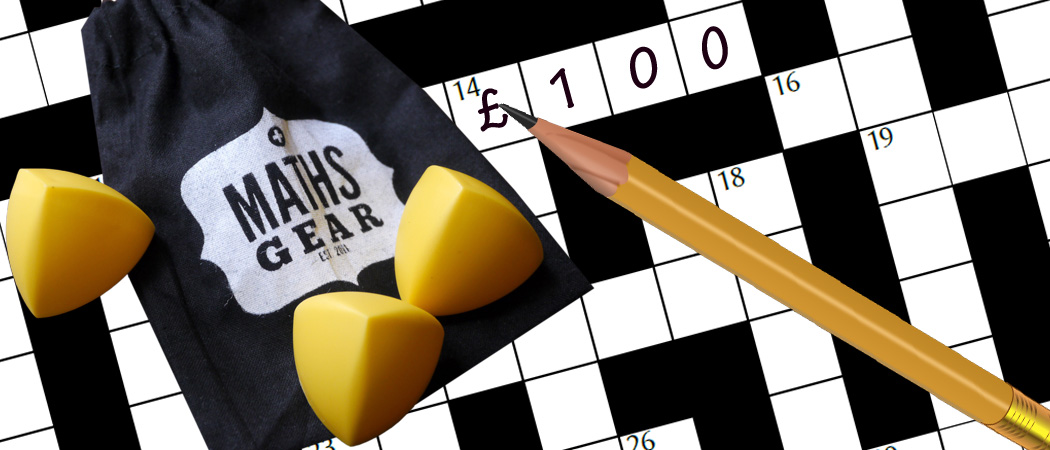# Prize crossnumber, Issue 08

Win £100 of Maths Gear goodies by solving our famously fiendish crossnumberOur original prize crossnumber is featured on pages 54 and 55 of Issue 08.

### Rules

• Although many of the clues have multiple answers, there is only one solution to the completed crossnumber. As usual, no numbers begin with 0. Use of Python, OEIS, Wikipedia, etc. is advised for some of the clues.
• One randomly selected correct answer will win a £100 Maths Gear goody bag, including non-transitive dice, a Festival of the Spoken Word DVD, a dodecaplex puzzle and much, much more. Three randomly selected runners up will win a Chalkdust T-shirt. The prizes have been provided by Maths Gear, a website that sells nerdy things worldwide. Find out more at mathsgear.co.uk
• To enter, submit the sum of the across clues via this form by 2 February 2019. Only one entry per person will be accepted. Winners will be notified by email and announced on our blog by 16 February 2019.

### Crossnumber

Crossnumber #8, set by Humbug:

### Clues

#### Across

• 1. The largest prime factor of one less than the square of this number is 3. (2)
• 3. The sum of this number’s digits is 18A. (8)
• 10. Every digit of this number is odd. (5)
• 12. The sum of 2D, 25D and 19A. (5)
• 13. A multiple of 1D whose first two digits are the same. (7)
• 15. Less than 1A. (2)
• 16. A palindrome. (7)
• 17. 20 more than 17D. (3)
• 18. A multiple of 3. (2)
• 19. A palindrome. (3)
• 20. This number and 17D are coprime. (4)
• 21. A prime number of the form $n^n+1$ for some integer $n$. (3)
• 22. This number and 20A are coprime. (3)
• 23. A prime number that is 16 more than 3 times 5D. (4)
• 25. A multiple of 10. (3)
• 26. Less than 1A. (2)
• 27. A factor of 24D. (3)
• 28. A seven-digit number. (7)
• 30. The highest common factor of 17A and 20A. (2)
• 31. A multiple of 32A. (7)
• 32. The first digit of this number is the first digit of 33D. (5)
• 34. A number $k$ such that $k\times2^n+1$ is not prime for any integer $n>0$. (5)
• 36. A prime number that is 16 more than 3 times 20D. (8)
• 37. Not a palindrome. (2)

#### Down

• 1. The first two digits of 11D. (2)
• 2. A palindrome. (5)
• 3. A prime number that is 16 more than 3 times 4D. (7)
• 4. A prime number that is 16 more than 3 times 22D. (6)
• 5. A prime number that is 16 more than 3 times 6D. (4)
• 6. A prime number. (3)
• 7. Greater than 1A. (2)
• 8. A prime number that is 16 more than 3 times 26D. (5)
• 9. A prime number that is 16 more than 3 times 36A. (8)
• 11. Less than 21D. (3)
• 14. The sum of this number’s factors (including 1 and the number itself) is three times this number. (3)
• 16. The sum of this number’s digits is 18A. (8)
• 17. This number and 22A are coprime. (3)
• 20. A prime number that is 16 more than 3 times 3D. (7)
• 21. A factor of 3A. (3)
• 22. A prime number that is 16 more than 3 times 8D. (6)
• 24. A multiple of 27A. (5)
• 25. A palindrome. (3)
• 26. A prime number that is 16 more than 3 times 23A. (5)
• 28. The largest prime factor of one less than the square of this number is 7. (4)
• 29. The sum of two consecutive square numbers. Also the sum of three consecutive square numbers. (3)
• 31. The largest prime factor of one less than the square of this number is 5. (3)
• 33. A multiple of 30A. (2)
• 35. An odd number that is not prime. (2)